### IMO Shortlist 2015 problem G3

Kvaliteta:
Avg: 0,0
Težina:
Avg: 7,0

Let$ABC$ be a triangle with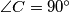$\angle{C} = 90^{\circ}$, and let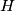$H$ be the foot of the altitude from$C$. A point$D$ is chosen inside the triangle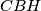$CBH$ so that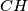$CH$ bisects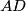$AD$. Let$P$ be the intersection point of the lines$BD$ and$CH$. Let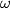$\omega$ be the semicircle with diameter$BD$ that meets the segment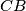$CB$ at an interior point. A line through$P$ is tangent to$\omega$ at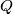$Q$. Prove that the lines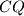$CQ$ and$AD$ meet on$\omega$.

(Georgia)

Izvor: https://www.imo-official.org/problems/IMO2015SL.pdf